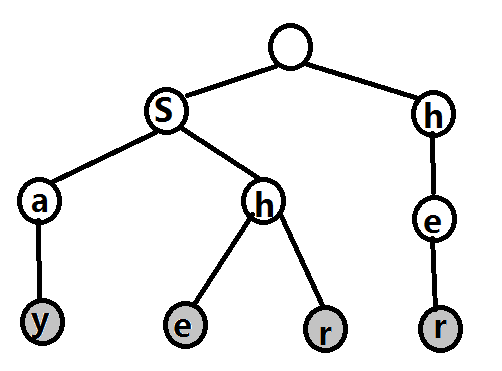# AC自动机

Trie（字典树）
KMP算法

（因为我默认你们上面的两个东西都学好了）

2. 求 aba aca bab sab sba 在字符串 asabbasbaabbabbacaacbscbs 中总共出现了几次。 嗯嗯嗯。。。 这个要怎么办？ 每次对一个需要匹配的串求一次next数组，然后一次次去匹配？ 显然，这样变得很慢很慢了。。。 如果需要匹配的串很多很多的话。。。。。 不敢想象。。，，

Aho-Corasick automation，该算法在1975年产生于贝尔实验室，是著名的多模匹配算法。

AC自动机需要提前知道所有的需要匹配的字符串

say she shr her（再说一遍，如果前面两个东西没学好，先去学习完再继续学习AC自动机）dalao们的blog告诉我们

Trie树的失配指针是指向：沿着其父节点 的 失配指针，一直向上，直到找到拥有当前这个字母的子节点 的节点 的那个子节点

void Get_fail()//构造fail指针
{
queue<int> Q;//队列
for(int i=0;i<26;++i)//第二层的fail指针提前处理一下
{
if(AC.vis[i]!=0)
{
AC[AC.vis[i]].fail=0;//指向根节点
Q.push(AC.vis[i]);//压入队列
}
}
while(!Q.empty())//BFS求fail指针
{
int u=Q.front();
Q.pop();
for(int i=0;i<26;++i)//枚举所有子节点
{
if(AC[u].vis[i]!=0)//存在这个子节点
{
AC[AC[u].vis[i]].fail=AC[AC[u].fail].vis[i];
//子节点的fail指针指向当前节点的
//fail指针所指向的节点的相同子节点
Q.push(AC[u].vis[i]);//压入队列
}
else//不存在这个子节点
AC[u].vis[i]=AC[AC[u].fail].vis[i];
//当前节点的这个子节点指向当
//前节点fail指针的这个子节点
}
}
}


AC自动机差不多就到这里

upd:2018.3.28

$$fail$$是失配指针，注意是失配

void BuildFail()
{
queue<int> Q;
for(it=t.son.begin();it!=t.son.end();++it)Q.push(it->second);
while(!Q.empty())
{
int u=Q.front();Q.pop();
if(!t[u].son.size())continue;
for(it=t[u].son.begin();it!=t[u].son.end();++it)
{
int v=it->second,c=it->first,p=t[u].ff;
while(p&&!t[p].son[c])p=t[p].ff;
if(t[p].son[c])t[v].ff=t[p].son[c];
Q.push(v);
t[v].fl|=t[t[v].ff].fl;
}
}
}

posted @ 2017-07-17 16:53  小蒟蒻yyb  阅读(31930)  评论(41编辑  收藏  举报